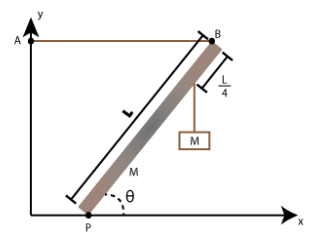# Problem: A uniform beam of length L and mass M has its lower end pivoted at P on the floor . making an angle θ with the floor. A horizontal cable is attached at its upper end B to a point A on a wall. A box of mass M is suspended from a rope that is attached to the beam one-fourth L from its upper end. Part (a) Write an expression for the y-component Py of the force exerted by the pivot on the bean. Part (b) Write an expression for the tension T in the horizontal cable AB.Part (c) Write an expression for the x-component Px of the force exerted by the pivot on the beam, in terms of T.Part (d) What is the tension in the horizontal cable, in newtons, if the mass of the beam is 38 kg, the length of the beam is 13 m, and the angle is 37°?

###### FREE Expert Solution

For a system in rotational equilibrium:

$\overline{){\mathbf{\Sigma }}{\mathbf{\tau }}{\mathbf{=}}{\mathbf{0}}}$

Torque:

$\overline{){\mathbf{\tau }}{\mathbf{=}}{\mathbf{r}}{\mathbf{F}}}$

(a)

We'll determine Py using the sum of vertical forces.

Vertical forces are Py, the weight of the box, and the weight of the beam.

Using Newton's second law:

ΣFy = ma

100% (185 ratings)###### Problem Details

A uniform beam of length L and mass M has its lower end pivoted at P on the floor . making an angle θ with the floor. A horizontal cable is attached at its upper end B to a point A on a wall. A box of mass M is suspended from a rope that is attached to the beam one-fourth L from its upper end.Part (a) Write an expression for the y-component Py of the force exerted by the pivot on the bean.

Part (b) Write an expression for the tension T in the horizontal cable AB.

Part (c) Write an expression for the x-component Px of the force exerted by the pivot on the beam, in terms of T.

Part (d) What is the tension in the horizontal cable, in newtons, if the mass of the beam is 38 kg, the length of the beam is 13 m, and the angle is 37°?